Which of the following compounds are not oxidized by HIO4?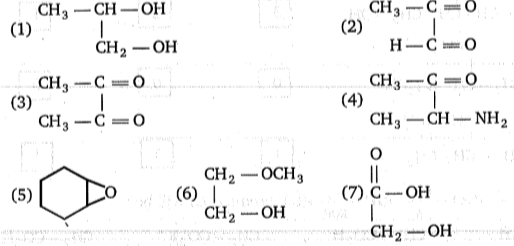(a) 5,6,7     (b) 4,5,6,7     (c) 6,7     (d) 3,4,5,6,7

Concept Questions :-

Ethers: Preparation, Physical & Chemical Properties, Uses
High Yielding Test Series + Question Bank - NEET 2020

Difficulty Level:

Succinic acid $\stackrel{∆}{\to }$(A)$\underset{∆}{\overset{{\mathrm{NH}}_{3}}{\to }}$(B)$\underset{\mathrm{KOH}}{\overset{{\mathrm{Br}}_{2}}{\to }}$(C); Product (C) will be:

(a) $\begin{array}{ccc}\underset{|}{{\mathrm{CH}}_{2}}& -& {\mathrm{CO}}_{2}\mathrm{H}\\ {\mathrm{CH}}_{2}& -& \begin{array}{cc}{\mathrm{CH}}_{2}& \begin{array}{cc}-& {\mathrm{NH}}_{2}\end{array}\end{array}\end{array}$

(b) $\begin{array}{ccc}\underset{|}{{\mathrm{CH}}_{2}}& -& {\mathrm{CO}}_{2}\mathrm{H}\\ {\mathrm{CH}}_{2}& -& {\mathrm{NH}}_{2}\end{array}$

(c) $\begin{array}{ccc}\underset{|}{{\mathrm{CH}}_{2}}& -& {{\mathrm{CO}}^{-}}_{2}{\mathrm{K}}^{+}\\ {\mathrm{CH}}_{2}& -& {\mathrm{NH}}_{2}\end{array}$

(d) $\begin{array}{ccc}\underset{|}{{\mathrm{CH}}_{2}}& -& {\mathrm{CO}}_{2}\mathrm{H}\\ {\mathrm{CH}}_{2}& -& \begin{array}{cc}{\mathrm{CH}}_{2}& \begin{array}{cc}-& \mathrm{Br}\end{array}\end{array}\end{array}$

Concept Questions :-

Alcohols: Preparation, Physical and Chemical Properties
High Yielding Test Series + Question Bank - NEET 2020

Difficulty Level: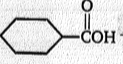L$\stackrel{{\mathrm{CH}}_{3}\mathrm{Li}}{\to }$M$\stackrel{{\mathrm{H}}_{3}{\mathrm{O}}^{+}}{\to }$ N + CH3OH

Product (N) is: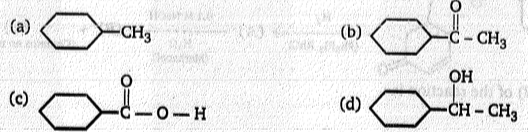Concept Questions :-

Alcohols: Preparation, Physical and Chemical Properties
High Yielding Test Series + Question Bank - NEET 2020

Difficulty Level: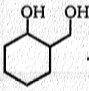$\stackrel{\mathrm{PCC}}{\to }$(A)$\underset{{\mathrm{H}}^{+}}{\overset{\mathrm{OH}-\mathrm{CH}2-\mathrm{CH}2-\mathrm{OH}}{\to }}$(B)(C)$\stackrel{{\mathrm{NaBH}}_{4}\mathrm{EtOH}}{\to }$(D) Product (D) in above reaction Is:

(a)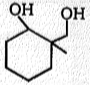(b)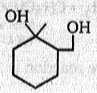(c)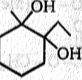(d)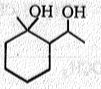High Yielding Test Series + Question Bank - NEET 2020

Difficulty Level:

(A) → B + C; (B) and (C) both gives +ve iodoform test.Compound (A) is:-
C5H10O       H3O+

(a) CH3-CH=CH-O-CH2-CH3

H
l
(b)CH3-C-O-CH2-CH3
l
CH3

(c)CH3-C-O-CH2-CH3
ll
CH2

(d)Both (b) and (c)

High Yielding Test Series + Question Bank - NEET 2020

Difficulty Level:

A solution of Ph3CCO2 in conc. H2SO4 gives (X) when poured into methanol X is :-
O
ll
(a) Ph3C-C-O-CH3

O
ll
(b) Ph2CH-C-O-CH3

(c) Ph3C-OCH3

(d) Ph3C-CH3

High Yielding Test Series + Question Bank - NEET 2020

Difficulty Level: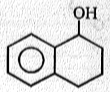$\underset{\mathrm{Pyridine}}{\overset{\mathrm{TsCl}}{\to }}$(A)$\stackrel{{\mathrm{LiAlH}}_{4}}{\to }$(B)

Product (B) of the above reaction is: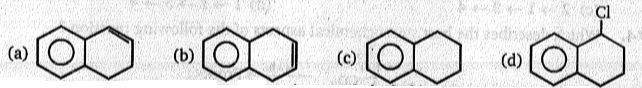High Yielding Test Series + Question Bank - NEET 2020

Difficulty Level:

Which of these is a reducing agent ?
1. CrO3/H+
2. KMnO4
3. LiAlH4
4. O3

Concept Questions :-

Alcohols: Preparation, Physical and Chemical Properties

Difficulty Level: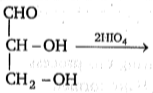Products obtained in the above reaction are :

(a) HCHO, HCO2H
(b) HCHO, 2HCO2H
(c) CO2, 2HCO2H
(d) CO2, HCHO, HCO2H

Concept Questions :-

Alcohols: Preparation, Physical and Chemical Properties
High Yielding Test Series + Question Bank - NEET 2020

Difficulty Level:

0.092 g of a compound with the molecular formula C3H8O reaction with an excess of CH3MgI gives 67.00 mL of methane at STP. The number of active hydrogen atoms present in a molecule of the compound is:
(a) one
(b) two
(c) three
(d) four

Concept Questions :-

Alcohols: Preparation, Physical and Chemical Properties